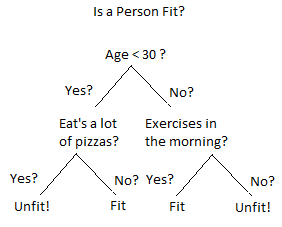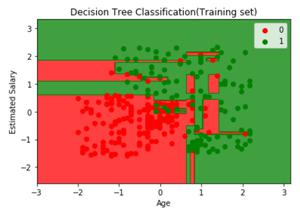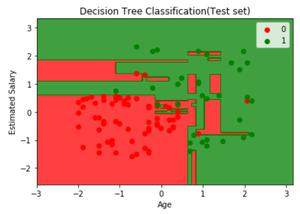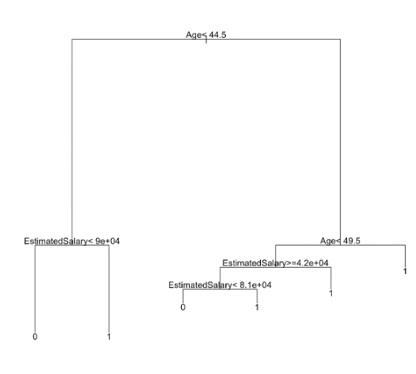Related Articles

# Decision Tree Classifiers in R Programming

• Difficulty Level : Medium
• Last Updated : 22 Jul, 2020

Classification is the task in which objects of several categories are categorized into their respective classes using the properties of classes. A classification model is typically used to,

• Predict the class label for a new unlabeled data object
• Provide a descriptive model explaining what features characterize objects in each class

There are various types of classification techniques such as,

### Decision Tree Classifiers

A decision tree is a flowchart-like tree structure in which the internal node represents feature(or attribute), the branch represents a decision rule, and each leaf node represents the outcome. A Decision Tree consists of,

• Nodes: Test for the value of a certain attribute.
• Edges/Branch: Represents a decision rule and connect to the next node.
• Leaf nodes: Terminal nodes that represent class labels or class distribution.And this algorithm can easily be implemented in the R language. Some important point about decision tree classifiers are,

• It is more interpretable
• Automatically handles decision-making
• Bisects the space into smaller spaces
• Prone to overfitting
• Can be trained on a small training set
• Majorly affected by noise

### Implementation in R

The Dataset:

A sample population of 400 people shared their age, gender, and salary with a product company, and if they bought the product or not(0 means no, 1 means yes). Download the dataset Advertisement.csv.

## R

 `# Importing the dataset``dataset = ``read.csv``(``'Advertisement.csv'``)``head``(dataset, 10)`

Output:

## R

 `# Encoding the target feature as factor``dataset\$Purchased = ``factor``(dataset\$Purchased, ``                           ``levels = ``c``(0, 1))`` ` `# Splitting the dataset into ``# the Training set and Test set``# install.packages('caTools')``library``(caTools)``set.seed``(123)``split = ``sample.split``(dataset\$Purchased, ``                     ``SplitRatio = 0.75)``training_set = ``subset``(dataset, split == ``TRUE``)``test_set = ``subset``(dataset, split == ``FALSE``)`` ` `# Feature Scaling``training_set[-3] = ``scale``(training_set[-3])``test_set[-3] = ``scale``(test_set[-3])`` ` `# Fitting Decision Tree Classification ``# to the Training set``# install.packages('rpart')``library``(rpart)``classifier = ``rpart``(formula = Purchased ~ .,``                   ``data = training_set)`` ` `# Predicting the Test set results``y_pred = ``predict``(classifier, ``                 ``newdata = test_set[-3], ``                 ``type = ``'class'``)`` ` `# Making the Confusion Matrix``cm = ``table``(test_set[, 3], y_pred)`
• The training set contains 300 entries.
• The test set contains 100 entries.
```Confusion Matrix:
[[62,  6],
[ 3, 29]]

```

Visualizing the Train Data:

## R

 `# Visualising the Training set results``# Install ElemStatLearn if not present ``# in the packages using(without hashtag)``# install.packages('ElemStatLearn')``library``(ElemStatLearn)``set = training_set`` ` `# Building a grid of Age Column(X1) ``# and Estimated Salary(X2) Column``X1 = ``seq``(``min``(set[, 1]) - 1, ``         ``max``(set[, 1]) + 1, ``         ``by = 0.01)``X2 = ``seq``(``min``(set[, 2]) - 1,``         ``max``(set[, 2]) + 1,``         ``by = 0.01)``grid_set = ``expand.grid``(X1, X2)`` ` `# Give name to the columns of matrix``colnames``(grid_set) = ``c``(``'Age'``,``                       ``'EstimatedSalary'``)`` ` `# Predicting the values and plotting them ``# to grid and labelling the axes``y_grid = ``predict``(classifier, ``                 ``newdata = grid_set,``                 ``type = ``'class'``)``plot``(set[, -3],``     ``main = 'Decision Tree ``             ``Classification ``(Training set)',``     ``xlab = ``'Age'``, ylab = ``'Estimated Salary'``,``     ``xlim = ``range``(X1), ylim = ``range``(X2))``contour``(X1, X2, ``matrix``(``as.numeric``(y_grid),``                       ``length``(X1),``                       ``length``(X2)), ``                       ``add = ``TRUE``)``points``(grid_set, pch = ``'.'``, ``       ``col = ``ifelse``(y_grid == 1, ``                    ``'springgreen3'``,``                    ``'tomato'``))``points``(set, pch = 21, bg = ``ifelse``(set[, 3] == 1, ``                                  ``'green4'``, ``                                  ``'red3'``))`

Output:Visualizing the Test Data:

## R

 `# Visualising the Test set results``library``(ElemStatLearn)``set = test_set`` ` `# Building a grid of Age Column(X1) ``# and Estimated Salary(X2) Column``X1 = ``seq``(``min``(set[, 1]) - 1, ``         ``max``(set[, 1]) + 1,``         ``by = 0.01)``X2 = ``seq``(``min``(set[, 2]) - 1,``         ``max``(set[, 2]) + 1,``         ``by = 0.01)``grid_set = ``expand.grid``(X1, X2)`` ` `# Give name to the columns of matrix``colnames``(grid_set) = ``c``(``'Age'``, ``                       ``'EstimatedSalary'``)`` ` `# Predicting the values and plotting them``# to grid and labelling the axes``y_grid = ``predict``(classifier,``                 ``newdata = grid_set,``                 ``type = ``'class'``)``plot``(set[, -3], main = 'Decision Tree ``                        ``Classification ``(Test set)',``     ``xlab = ``'Age'``, ylab = ``'Estimated Salary'``,``     ``xlim = ``range``(X1), ylim = ``range``(X2))``contour``(X1, X2, ``matrix``(``as.numeric``(y_grid),``                       ``length``(X1), ``                       ``length``(X2)),``                       ``add = ``TRUE``)``points``(grid_set, pch = ``'.'``, ``       ``col = ``ifelse``(y_grid == 1, ``                    ``'springgreen3'``,``                    ``'tomato'``))``points``(set, pch = 21, bg = ``ifelse``(set[, 3] == 1,``                                  ``'green4'``,``                                  ``'red3'``))`

Output:Decision Tree Diagram:

## R

 `# Plotting the tree``plot``(classifier)``text``(classifier)`

Output:Attention reader! Don’t stop learning now. Get hold of all the important Machine Learning Concepts with the Machine Learning Foundation Course at a student-friendly price and become industry ready.

My Personal Notes arrow_drop_up